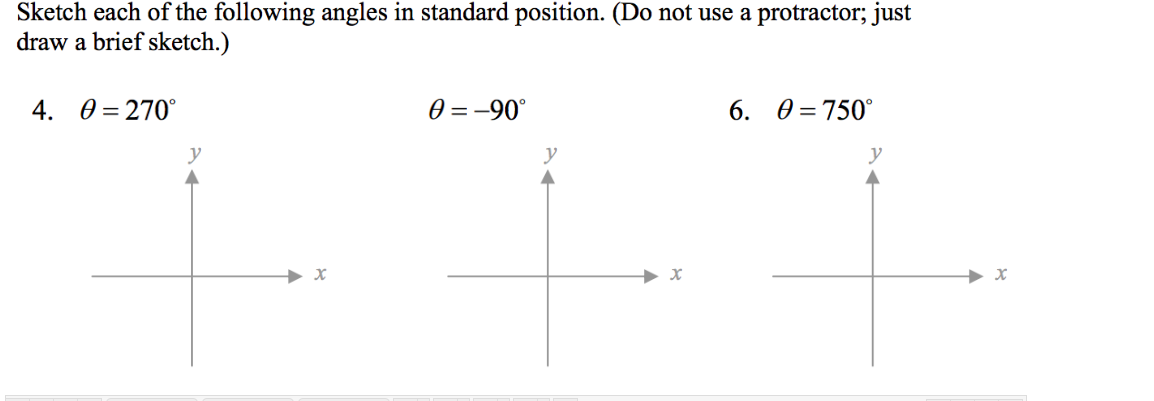# Sketch each of the following angles in standard position. (Do not use a protractor; justdraw a brief sketch.)0 = 270°0 = -90°6. 0 =750°4.

Question
2 viewshelp_outlineImage TranscriptioncloseSketch each of the following angles in standard position. (Do not use a protractor; just draw a brief sketch.) 0 = 270° 0 = -90° 6. 0 =750° 4. fullscreen
check_circle

Step 1

To sketch the angles in the standand position.

Step 2

Given:

Step 3

Part (4)

The coordinate axis is at right angle to each other.

Since, the angle 270 = 3 right angles so s...

### Want to see the full answer?

See Solution

#### Want to see this answer and more?

Solutions are written by subject experts who are available 24/7. Questions are typically answered within 1 hour.*

See Solution
*Response times may vary by subject and question.
Tagged in

### Other